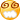## 12 条留言  访客：12 条  博主：0 条

1.fhjshr。错了5题，有不会的但是也有看错题或者做错的。要哭了

2.blue_snow

17题：（已知y=ax^7 bx^5 cx^3 dx e，其中a,b,c,d,e为常数，当x=2时，y=23,当x=-2时，y=-35,求|e|=?）是个有问题的题，根据题意常规思路马上可以得出2e=-12,所以|e|=6.但是，无论e=6或e=-6，都是偶数，偶数 2n不能得出23，偶数-2n也不能得出-35，因此与题干矛盾，无解。

3.blue_snow

17题：（已知y=ax^7+bx^5+cx^3+dx+e，其中a,b,c,d,e为常数，当x=2时，y=23,当x=-2时，y=-35,求|e|=?）是个有问题的题，根据题意常规思路马上可以得出2e=-12,所以|e|=6.但是，无论e=6或e=-6，都是偶数，偶数+2n不能得出23，偶数-2n也不能得出-35，因此与题干矛盾，无解。

4.morgandy

我晕了，为什么下载下答案跟子龙同学的提供的答案不同？如7、9，我做的答案跟子龙一样。

5.rickyincufe

和以上各位的感觉一样  一看这三十道题 没有一道不会做的 但是还是错了好几道  不是少看一个数  就是在草纸上写的1 抄到答案就变成-1了 看来 所谓的简单题 并不简单啊

6.黄英

绝对值检查增根,还是有些糊涂

7.tina0205

陈老师答案中有一些问题：
第二题题目中最后求的应该是是m的值，并且答案应该选A。
第九题，题目中（1）如果求的是整数的值，答案应该是A、B.将C选项回带题中等式不成立。（2）如果题目中求的是整数值的个数。那么答案选择C（两个值是0和1）.

8.roamok

与 子龙—Jalen 有同感——
“尤其是简单题，或者见过的题，错误率更高。
好像遇到这种题，脑子就不转了似的，最后不是审题就是计算错。这种错误比不会做感觉更可惜。”
郁闷中…………

9.子龙-Jalen

陈老师，很郁闷，现在发现很多题会做，做题速度也过得去，但总会做错。

尤其是简单题，或者见过的题，错误率更高。

好像遇到这种题，脑子就不转了似的，直接跳过去了，最后不是审题就是计算错。这种错误比不会做感觉更可惜。

正式考试时，因为紧张，可能更容易犯这样的错误，很是担心。

10.子龙-Jalen

01. -10 (去增根0)

02. A

03. 11 (去增根-1/11)

04. -5, -1, 3 (法1：分段， 法2：图像)

05. D

06. C (画数轴，三点中间最大也不可能，两边各一个)

07. B (画数轴, -3,-2,-1,0)

08. b<=x<=a (特值或|a-x| |x-b|=a-b在(a-x)(x-b)>=0时成立)

09. AB

10. 1<=x<=5

11. 3, 7

12. 8 (xy y=(x 1)y=(6-y)y=-y^2 6y=-(y-3)^2 9<=9, so y=3, x=2, z=0)

13. 0 (x=0)

14. B

15. C ((x-y)^2=x^2 y^2-2xy=16, (|x| |y|)^2=x^2 y^2 2|xy|=49 => |xy| xy>0 => xy>0 => (x y)^2=(|x| |y|)^2=49)

16. D (不同正整数 => 7-x必不同 => (7-m)(…)必为-1,1,-2,2 => 5,6,8,9)

17. 6

18. E

19. -3

20. 0

21. -2

22. (-1,0), (0,-1), (1,0), (0,1), (1,-1), (-1,1)

23. [1/3,4/5], 9 (4-5x>=0, 1-3x<=0)

24. a>=1 (图像)

25. c

26. d,a,c,b (1说明bd一个最大一个最小, 2说明d-a和a-c同号, c第2大说明a-c<0, d-a<0, 则d<a<c<b)

27. x 1 (-1<=x<=1)

28. 3, -1

29. -1 (原式=-(a b c) (b-c) a-(c 1)=-3c-1)

30. 15 (原式=x-p 15-x 15 p-x=30-x)

11.子龙-Jalen

01. -10 (去增根0)

02. A

03. 11 (去增根-1/11)

04. -5, -1, 3 (法1：分段， 法2：图像)

05. D

06. C (画数轴，三点中间最大也不可能，两边各一个)

07. B (画数轴, -3,-2,-1,0)

08. b<=x<=a (特值或|a-x|+|x-b|=a-b在(a-x)(x-b)>=0时成立)

09. AB

10. 1<=x<=5

11. 3, 7

12. 8 (xy+y=(x+1)y=(6-y)y=-y^2+6y=-(y-3)^2+9<=9, so y=3, x=2, z=0)

13. 0 (x=0)

14. B

15. C ((x-y)^2=x^2+y^2-2xy=16, (|x|+|y|)^2=x^2+y^2+2|xy|=49 => |xy|+xy>0 => xy>0 => (x+y)^2=(|x|+|y|)^2=49)

16. D (不同正整数 => 7-x必不同 => (7-m)(…)必为-1,1,-2,2 => 5,6,8,9)

17. 6

18. E

19. -3

20. 0

21. -2

22. (-1,0), (0,-1), (1,0), (0,1), (1,-1), (-1,1)

23. [1/3,4/5], 9 (4-5x>=0, 1-3x<=0)

24. a>=1 (图像)

25. c

26. d,a,c,b (1说明bd一个最大一个最小, 2说明d-a和a-c同号, c第2大说明a-c<0, d-a<0, 则d<a<c<b)

27. x+1 (-1<=x<=1)

28. 3, -1

29. -1 (原式=-(a+b+c)+(b-c)+a-(c+1)=-3c-1)

30. 15 (原式=x-p+15-x+15+p-x=30-x)

12.定定儿

谢谢陈老师！